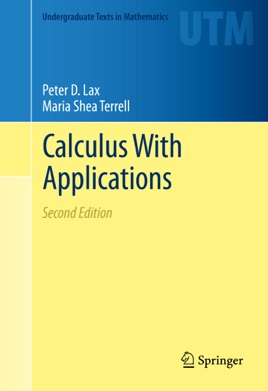• 35,99 €

## Descrizione dell’editore

This new edition of Lax, Burstein, and Lax's Calculus with Applications and Computing offers meaningful explanations of the important theorems of single variable calculus. Written with students in mathematics, the physical sciences, and engineering in mind, and revised with their help, it shows that the themes of calculation, approximation, and modeling are central to mathematics and the main ideas of single variable calculus. This edition brings the innovation of the first edition to a new generation of students. New sections in this book use simple, elementary examples to show that when applying calculus concepts to approximations of  functions, uniform convergence is more natural and easier to use than point-wise convergence. As in the original, this edition includes material that is essential for students in science and engineering, including an elementary introduction to complex numbers and complex-valued functions, applications of calculus to modeling vibrations and population dynamics, and an introduction to probability and information theory.